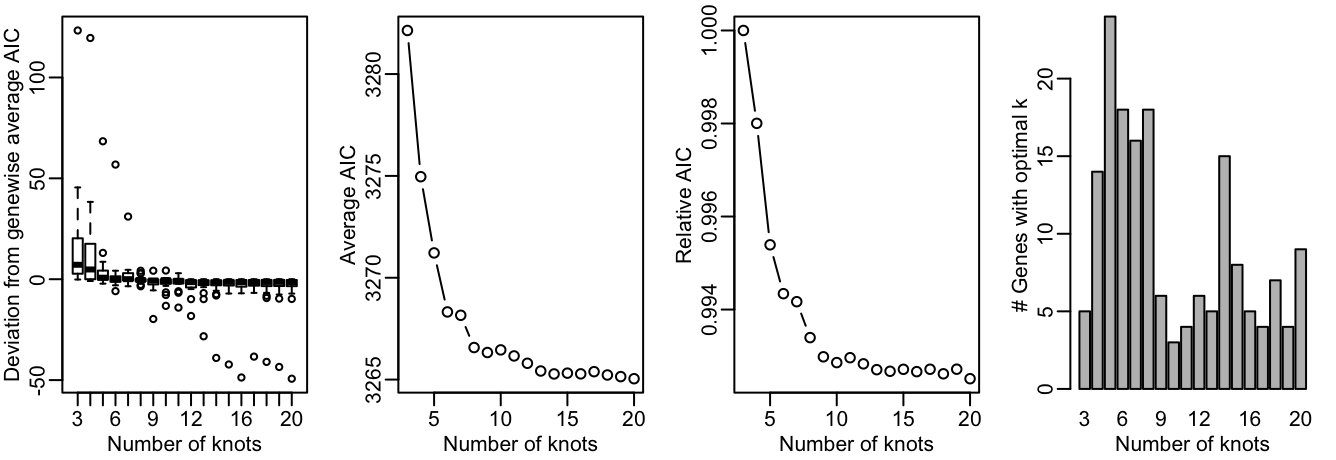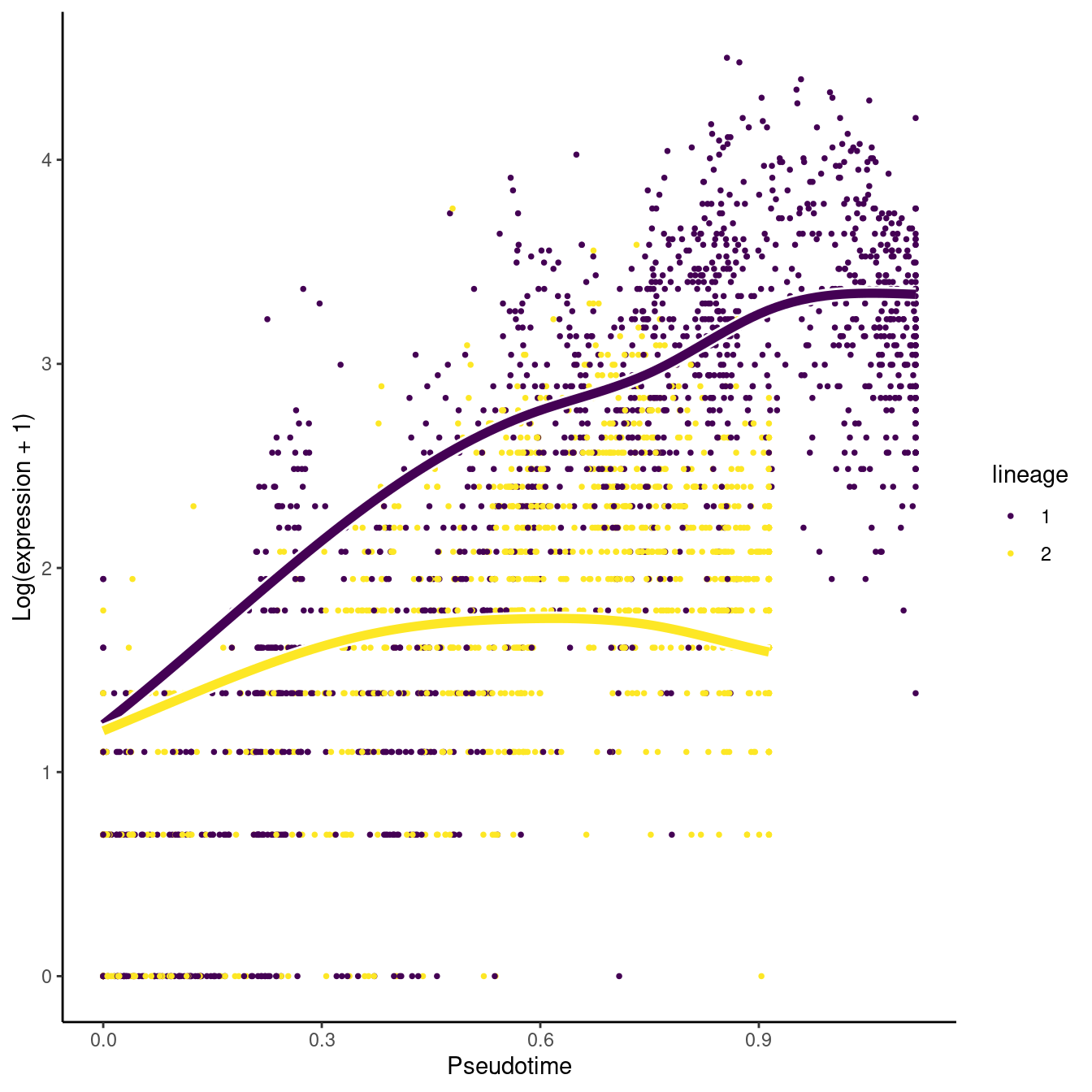`tradeSeq` is an `R` package that allows analysis of gene expression along trajectories. While it has been developed and applied to single-cell RNA-sequencing (scRNA-seq) data, its applicability extends beyond that, and also allows the analysis of, e.g., single-cell ATAC-seq data along trajectories or bulk RNA-seq time series datasets. For every gene in the dataset, `tradeSeq` fits a generalized additive model (GAM) by building on the `mgcv` R package. It then allows statistical inference on the GAM by assessing contrasts of the parameters of the fitted GAM model, aiding in interpreting complex datasets. All details about the `tradeSeq` model and statistical tests are described in our preprint (Van den Berge et al. 2020).

In this vignette, we analyze a subset of the data from (Paul et al. 2015). A `SingleCellExperiment` object of the data has been provided with the `tradeSeq` package and can be retrieved as shown below. The data and UMAP reduced dimensions were derived from following the Monocle 3 vignette.

In this vignette, we use `tradeSeq` downstream of `slinghsot`(Street et al. 2018) but it can be used downstream of any trajectory. In particular, if you want to use `tradeSeq` downstream of `monocle3`(Cao et al. 2019), please refer to our Monocle vignette.

# Installation

To install the package, simply run

``````if(!requireNamespace("BiocManager", quietly = TRUE)) {
install.packages("BiocManager")
}

``````library(tradeSeq)
library(RColorBrewer)
library(SingleCellExperiment)
library(slingshot)

# For reproducibility
RNGversion("3.5.0")
palette(brewer.pal(8, "Dark2"))
counts <- as.matrix(countMatrix)
rm(countMatrix)

# Fit negative binomial model

Here we fit the `tradeSeq` negative binomial generalized additive model (NB-GAM). Please see the fitGAM vignette for an extensive description on how to fit the models, tune its options and modify its output.

We first need to decide on the number of knots. This is done using the evaluateK function. This takes a little time to run so it is not run here.

``````set.seed(5)
icMat <- evaluateK(counts = counts, sds = crv, k = 3:10,
nGenes = 200, verbose = T)``````For more explanation on the output from evaluateK, we refer users to the fitGAM vignette. Here, we pick `nknots = 6`.

We then fit the models by running the fitGAM function. By default, the gene-wise NB-GAM estimates one smoother for every lineage using the negative binomial distribution. Please refer to the fitGAM vignette Additional to add additional covariates to the model, speed up computation or allow for custom normalization, amongst others.

``````set.seed(7)
pseudotime <- slingPseudotime(crv, na = FALSE)
cellWeights <- slingCurveWeights(crv)
sce <- fitGAM(counts = counts, pseudotime = pseudotime, cellWeights = cellWeights,
nknots = 6, verbose = FALSE)``````

The model may be hard to fit for some genes, and hence the fitting procedure may not converge for all of the genes in a dataset, especially in datasets with complex patterns and/or many lineages. You can check the convergence of each gene as shown below, where a `TRUE` value corresponds to a converged model fit, and a `FALSE` value corresponds to a model that hasn’t been able to converge fully.

``table(rowData(sce)\$tradeSeq\$converged)``
``````##
## TRUE
##  240``````

# Within-lineage comparisons

## Association of gene expression with pseudotime

A first exploration of the data analysis may consist of checking whether gene expression is associated with a particular lineage. The statistical test performed here, implemented in the `associationTest` function, is testing the null hypothesis that all smoother coefficients are equal to each other. This can be interpreted as testing whether the average gene expression is significantly changing along pseudotime.

``````assoRes <- associationTest(sce)
``````##        waldStat df       pvalue meanLogFC
## Acin1        NA NA           NA 0.3155838
## Actb  455.84722 10 0.000000e+00 0.5610723
## Ak2    87.90779 10 1.387779e-14 0.7030388
## Alad         NA NA           NA 1.0476606
## Alas1 748.31595 10 0.000000e+00 1.1210974
## Aldoa   0.00000 10 1.000000e+00 0.4340672``````

## Discovering progenitor marker genes

In order to discover marker genes of the progenitor or differentiated cell population, researchers may be interested in assessing differential expression between the progenitor cell population (i.e., the starting point of a lineage) with the differentiated cell type population (i.e., the end point of a lineage). The function `startVsEndTest` uses a Wald test to assess the null hypothesis that the average expression at the starting point of the smoother (progenitor population) is equal to the average expression at the end point of the smoother (differentiated population). The test basically involves a comparison between two smoother coefficients for every lineage. The function `startVsEndTest` performs a global test across all lineages by default (i.e. it compares the start and end positions for all lineages simultaneously), but you can also assess all lineages separately by setting `lineages=TRUE`. Below, we adopt an omnibus test across the two lineages.

``startRes <- startVsEndTest(sce)``

We can visualize the estimated smoothers for the third most significant gene.

``````oStart <- order(startRes\$waldStat, decreasing = TRUE)
sigGeneStart <- names(sce)[oStart]
plotSmoothers(sce, counts, gene = sigGeneStart)``````Alternatively, we can color the cells in UMAP space with that gene’s expression.

``plotGeneCount(crv, counts, gene = sigGeneStart)``